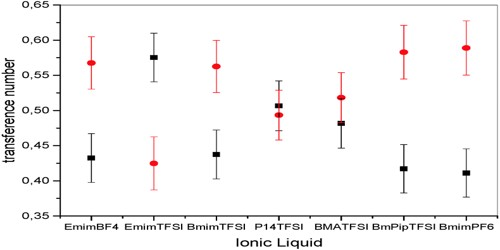# Abnormal Transference Number

Abnormal Transference number

It is sometimes found that the transport numbers of some ions decrease rapidly with an increase in the concentration of the ions. Examples are the transport number of silver in ammoniacal solution, cadmium in cadmium iodide. This change in transport number is attributed to complex formation, whereby the metallic ion forms a part of a complex anion and travels to the anode. The fraction of total electric current carried by the ions or ionic species present in an electrolyte is known as transport number. For cadmium this is represented as follows:

2Cdl2 ↔ Cd2+ + [Cdl4]2-

Mathematically, transport no. for cation = v+ / (v+ + v)

where v+ = speed of migration of cation,

v = speed of migration of anion,

v+ + v = 1 always.

It is apparent that the transference number or transport number has to lie between and 1, otherwise, the positive ion would be traveling alongside the current or the negative ion with the current, and this is inconceivable. A transport number must lie between 0 and 1 otherwise, it means that the positive ion is moving against the current or the negative ion against the current. Nevertheless, Hittorf found for the transference number or transport number of iodine in a 4*8 percent solution of cadmium iodide in- alcohol the value 2*1, and in a 3 percent, solution the value 1*3. At a very high dilution, the value would probably sink below 1, i,e. would lose its abnormality. This abnormal behavior of the ions is known as an abnormal transport number.